Next: Exponential growth and decay Up: Background Previous: Background

### Exponential functions in Maple

An exponential function is a function of the formwhere b is a positive constant and x is any real number. The number b is often called the base. The special case b=1 is usually excluded, because that would just give the constant function f(x)=1.

Defining an exponential function in Maple is straightforward. For example, to define and plot the exponential function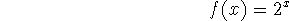the following Maple commands could be used. They also show how to obtain numerical values for several x values.

```  > f := x -> 2^x;
```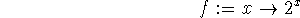```  > f(0);
```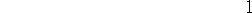```  > f(2.5);
```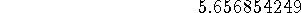```  > f(Pi);
```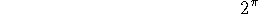```  > evalf(f(Pi));
```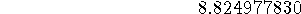```  > plot(f(x),x=-3..3);
```

The behavior of an exponential function depends very much on whether the base, b is smaller or larger than 1. For example, look at the plot generated by the following command. Make sure you understand which curve is which, and how these two curves are related.

```  > plot({2^x,(1/2)^x},x=-3..3);
```

A problem that often arises in applications is to fit data to an exponential function. For example, suppose you knew that g(x) was an exponential function and that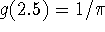. You can use this information to solve for the value of b, because it must satisfy the equation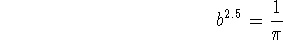In Maple, this can be done with the solve or fsolve command. The following example shows how to find the value of b, and use it to construct the function g(x). Notice the use of the label b1 in the definition of the function.

```  > b1 := solve(b^2.5 = 1/Pi,b);
``````  > g := x -> b1^x;
``````  > g(2.5);
```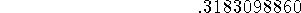```  > plot(g(x),x=0..6);
```

You might ask why it wouldn't have been simpler to just use b for the label. The answer is that this would have worked the first time you executed the command, but would generate an error the second time you tried it, as shown below.

```  > b := solve(b^2.5 = 1/Pi,b);
```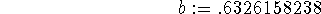```  > b := solve(b^2.5 = 1/Pi,b);
```

```Error, (in solve) a constant is invalid as a variable, .6326158238

```

The problem the second time is that the label b has a value after the first command above, so it can't act as a variable in the second command.

The conclusion you should draw here is the following. If you need to solve an equation in Maple and want to label the result so you can use it later, don't use the same name for the variable in the equation and the label. Before you go on, you should clear out the value of b with the command below. This makes b back into a variable.

```  > b := 'b';
```Next: Exponential growth and decay Up: Background Previous: Background

William W. Farr
Fri Jan 31 14:58:36 EST 1997